#8个Python高效数据分析的技巧

0
0
0## 8个Python高效数据分析的技巧

Python for Data Science: 8 Concepts
You May Have Forgotten

x = [1,2,3,4]
out = []
for item in x:
out.append(item**2)
print(out)
[1, 4, 9, 16]
# vs.
x = [1,2,3,4]
out = [item**2 for item in x]
print(out)
[1, 4, 9, 16]


Lambda表达式

lambda表达式的基本语法是：

lambda arguments: expression


double = lambda x: x * 2
print(double(5))
10


Map和Filter

# Map
seq = [1, 2, 3, 4, 5]
result = list(map(lambda var: var*2, seq))
print(result)
[2, 4, 6, 8, 10]


Filter函数接受一个列表和一条规则，就像map一样，但它通过比较每个元素和布尔过滤规则来返回原始列表的一个子集。

# Filter
seq = [1, 2, 3, 4, 5]
result = list(filter(lambda x: x > 2, seq))
print(result)
[3, 4, 5]


Arange和Linspace

Arange返回给定步长的等差列表。 它的三个参数start、stop、step分别表示起始值，结束值和步长， 请注意，stop点是一个“截止”值，因此它不会包含在数组输出中。

# np.arange(start, stop, step)
np.arange(3, 7, 2)
array([3, 5])


Linspace和Arrange非常相似，但略有不同。 Linspace以指定数目均匀分割区间。 所以给定区间start和end，以及等分分割点数目num，linspace将返回一个NumPy数组。 这对绘图时数据可视化和声明坐标轴特别有用。

# np.linspace(start, stop, num)
np.linspace(2.0, 3.0, num=5)
array([ 2.0,  2.25,  2.5,  2.75, 3.0])


Axis代表什么？

df.drop('Column A', axis=1)
df.drop('Row A', axis=0)


df.shape
(# of Rows, # of Columns)


Concat，Merge和Join

Concat允许用户在表格下面或旁边追加一个或多个DataFrame（取决于您如何定义轴）。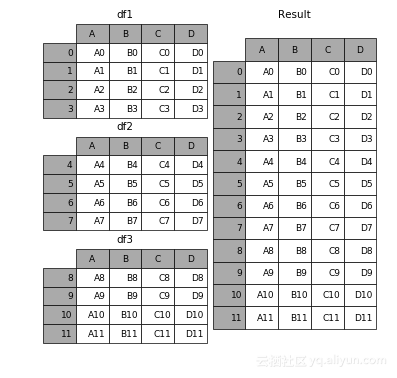Merge将多个DataFrame合并指定主键（Key）相同的行。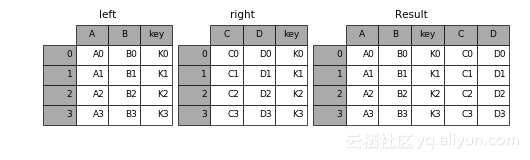Join，和Merge一样，合并了两个DataFrame。 但它不按某个指定的主键合并，而是根据相同的列名或行名合并。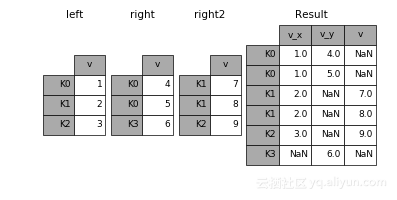Pandas Apply

Apply是为Pandas Series而设计的。如果你不太熟悉Series，可以将它想成类似Numpy的数组。

Apply将一个函数应用于指定轴上的每一个元素。 使用Apply，可以将DataFrame列（是一个Series）的值进行格式设置和操作，不用循环，非常有用！

df = pd.DataFrame([[4, 9],] * 3, columns=['A', 'B'])
df
A  B
0  4  9
1  4  9
2  4  9

df.apply(np.sqrt)
A    B
0  2.0  3.0
1  2.0  3.0
2  2.0  3.0

df.apply(np.sum, axis=0)
A    12
B    27

df.apply(np.sum, axis=1)
0    13
1    13
2    13


Pivot Tables

pd.pivot_table(df, index=["Manager", "Rep"])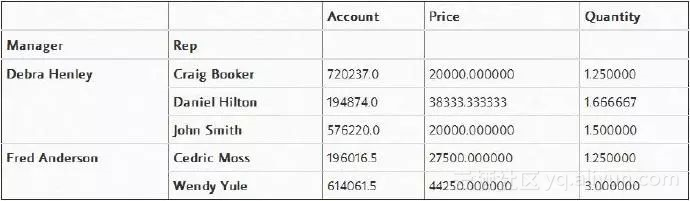pd.pivot_table(df,index=["Manager","Rep"],values=["Price"])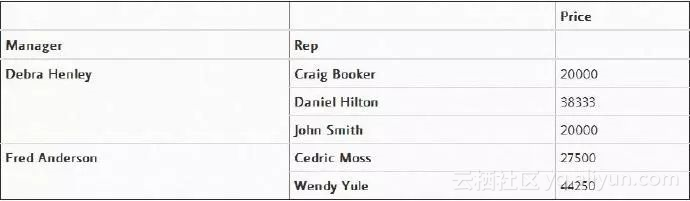+ 关注